Introduction

When you are configuring for integration, there may be a need to create an array with configurable start and end limits

For example, you want to create an array with the objects has id property starting from 4 to 7 through its objects, such as below:

```[
{
"id": 4
},
{
"id": 5
},
{
"id": 6
},
{
"id": 7
}
] ```

In this guide, we will show you how to do it within an entity transformer configuration.

Steps

1. First, you need to have the limits (start and end) in the entity data or set them yourself. Example:
```{
"start": 4,
"end": 7
} ```

2. It’s recommended that you use the Chain multiple entity transformers as you will put more than one entity transformers if you decide to create it in a single entity transformer.
3. Then, inside a Data, transform data using mappers and conditions, you need to subtract the end limit by the start limit using a Value Setter and an Operator mapper (Subtraction) to get the difference. If you want to create the array from 4 to 7, you need to add the Prepared Operator mapper (Addition) and set the Left to 1 (number).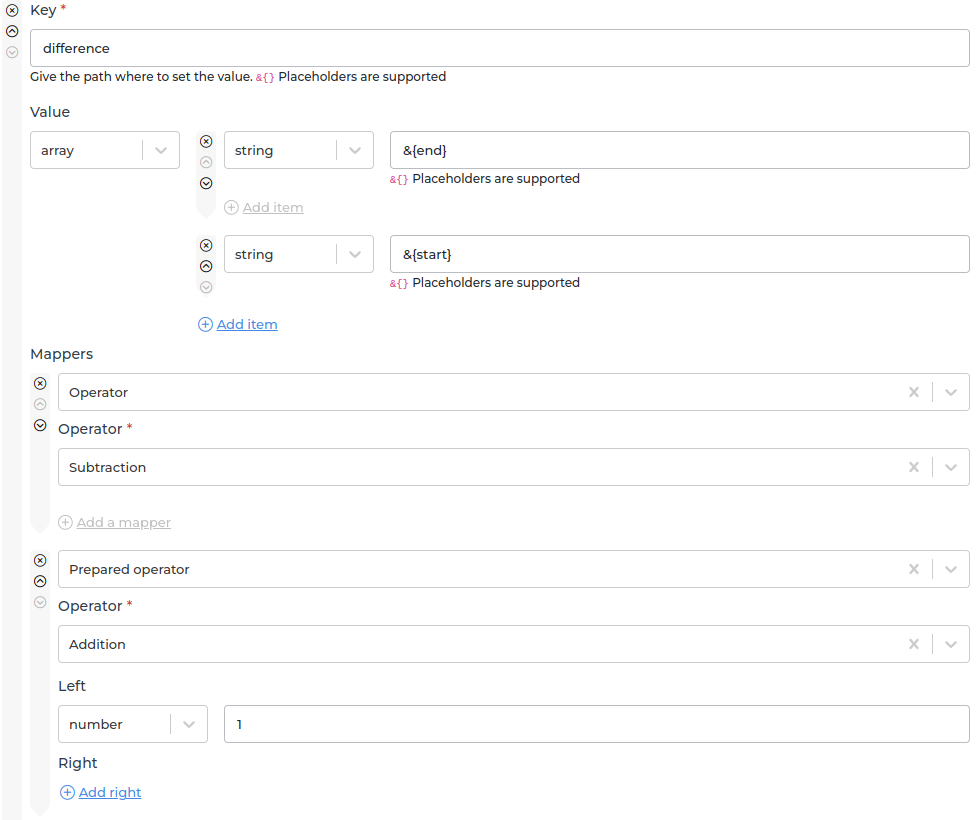4. Next, after getting the difference, it should be converted to an array where the length equals to the difference using List: Fill list with value mapper and set the Length to &{@}, which represents the current value of the difference property. The result is an array of integers, i.e., [“4”, “4”, “4”, “4”] when the difference is 4.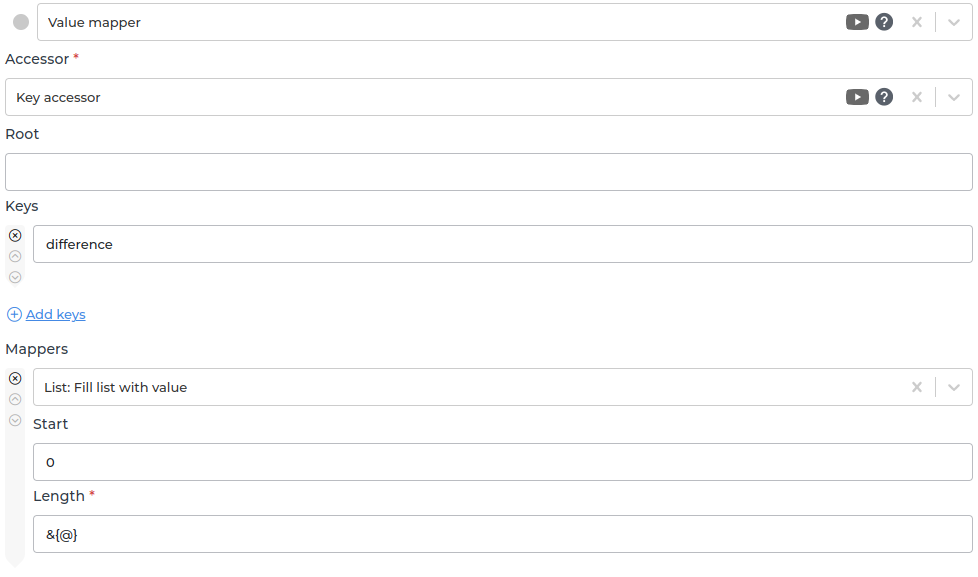5. Then, the array should be converted to an array of objects using the Move data between accessor. The Source accessor is a Key accessor, and the Destination accessor is the Structure accessor. So, the array would be something like this:
```{
"difference": [
{
"index": "0",
"value": 3
},
{
"index": "1",
"value": 3
},
{
"index": "2",
"value": 3
}
]
}```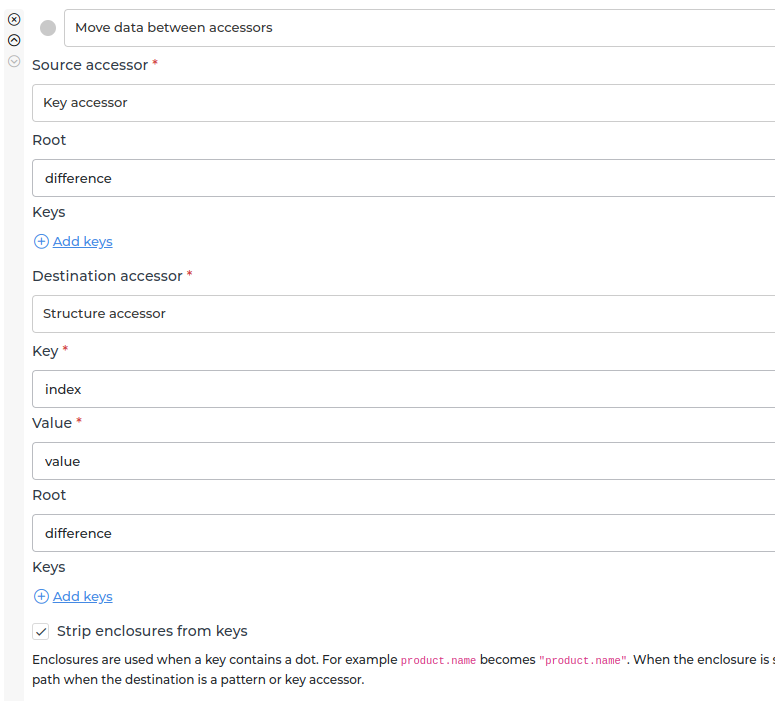6. Then, we can copy the start limit to all the objects using the Recursively copy values to children transformer so that every object will have the start limit, such as below.
```{
"difference": [
{
"index": "0",
"value": 3,
"start": 4
},
{
"index": "1",
"value": 3,
"start": 4
},
{
"index": "2",
"value": 3,
"start": 4
}
]
}```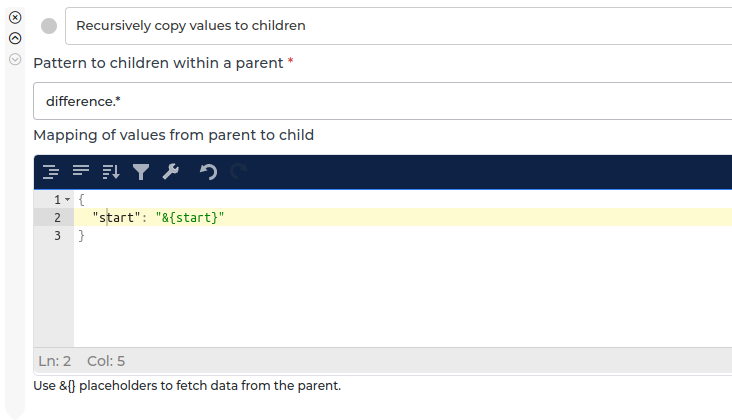7. Then, inside a Node, transform nodes, you need to create a new attribute, for example, id, where the value is the sum of the index and start values.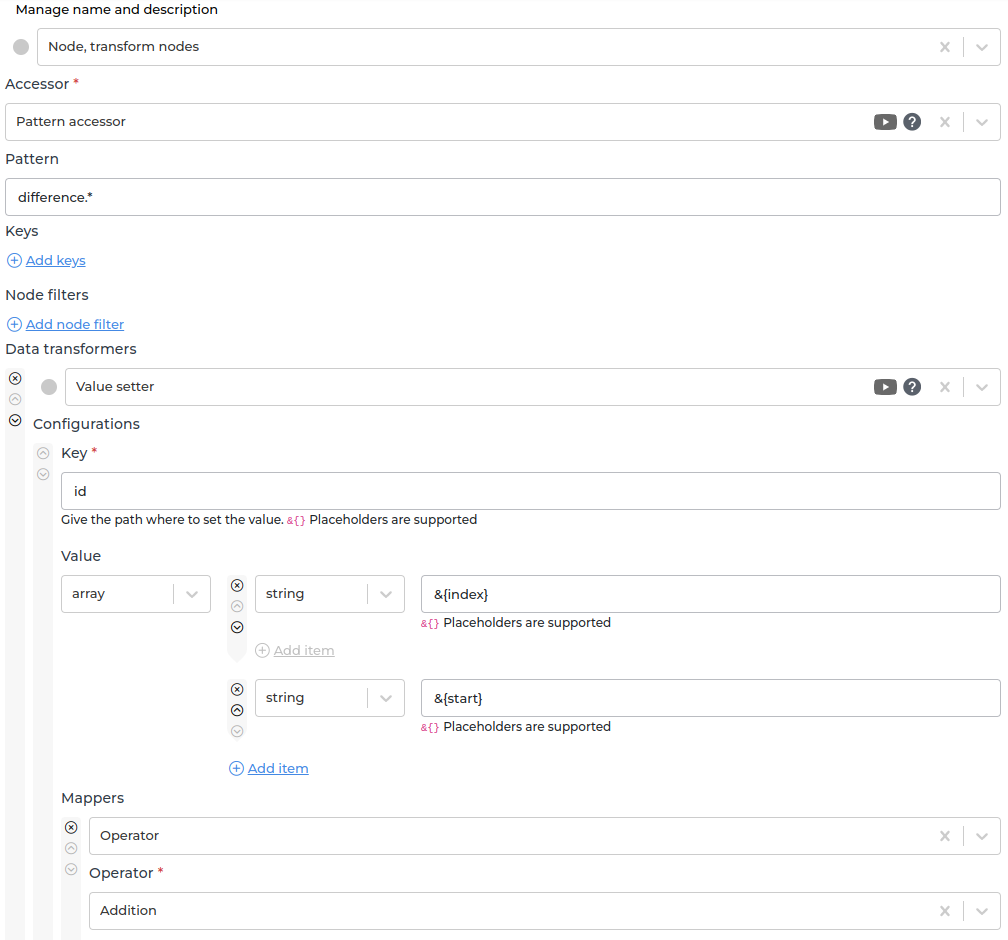8. Then you can remove other attributes, except id, using the Key Filter.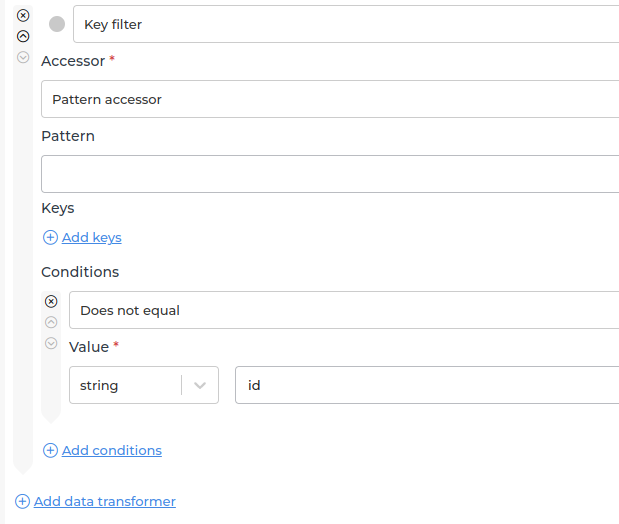9. Finally, in the data transformer (outside the node) use the List Mapper and the Get values mapper to tidy up the data structure.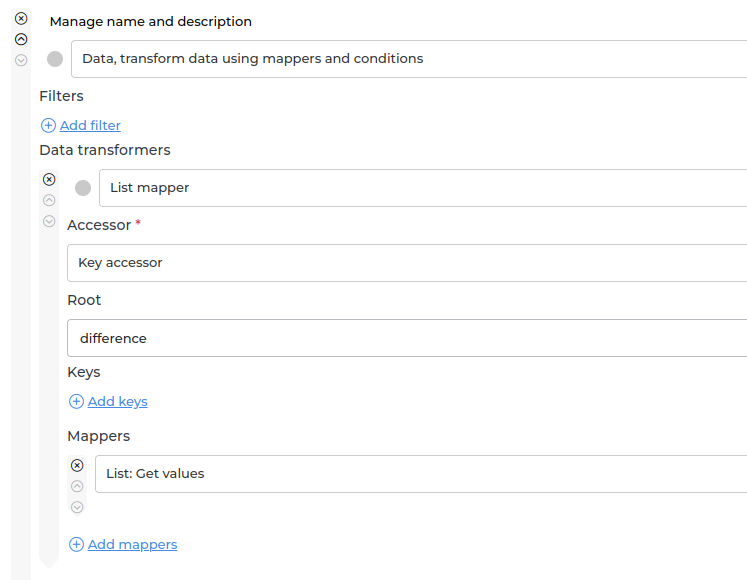And here is the final result.

```{
"start": 4,
"end": 7,
"difference": [
{
"id": 4
},
{
"id": 5
},
{
"id": 6
},
{
"id": 7
}
]
} ```